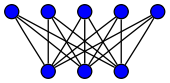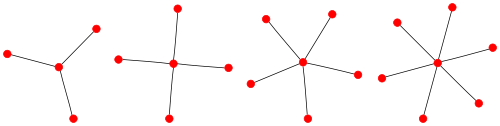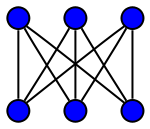# Complete bipartite graph

﻿
Complete bipartite graph
Complete bipartite graphA complete bipartite graph with m = 5 and n = 3
Vertices n + m
Edges mn
Girth 4
Automorphisms 2m!n! if m=n, otherwise m!n!
Chromatic number 2
Chromatic index max{m, n}
Notation Km,n
v · mathematical field of graph theory, a complete bipartite graph or biclique is a special kind of bipartite graph where every vertex of the first set is connected to every vertex of the second set.

## Definition

A complete bipartite graph, G := (V1 + V2, E), is a bipartite graph such that for any two vertices, v1V1 and v2V2, v1v2 is an edge in G. The complete bipartite graph with partitions of size |V1|=m and |V2|=n, is denoted Km,n.

## ExamplesThe star graphs S3, S4, S5 and S6.The utility graph K3,3
• For any k, K1,k is called a star. All complete bipartite graphs which are trees are stars.
• The graph K1,3 is called a claw, and is used to define the claw-free graphs.
• The graph K3,3 is called the utility graph. This usage comes from a standard mathematical puzzle in which three utilities must each be connected to three buildings; it is impossible to solve without crossings due to the nonplanarity of K3,3.

## Properties

• Given a bipartite graph, finding its complete bipartite subgraph Km,n with maximal number of edges mn is an NP-complete problem.
• A planar graph cannot contain K3,3 as a minor; an outerplanar graph cannot contain K3,2 as a minor (These are not sufficient conditions for planarity and outerplanarity, but necessary).
• A complete bipartite graph. Kn,n is a Moore graph and a (n,4)-cage.
• A complete bipartite graph Kn,n or Kn,n+1 is a Turán graph.
• A complete bipartite graph Km,n has a vertex covering number of min{m,n} and an edge covering number of max{m,n}.
• A complete bipartite graph Km,n has a maximum independent set of size max{m,n}.
• The adjacency matrix of a complete bipartite graph Km,n has eigenvalues √(nm), −√(nm) and 0; with multiplicity 1, 1 and n+m−2 respectively.
• The laplacian matrix of a complete bipartite graph Km,n has eigenvalues n+m, n, m, and 0; with multiplicity 1, m−1, n−1 and 1 respectively.
• A complete bipartite graph Km,n has mn−1 nm−1 spanning trees.
• A complete bipartite graph Km,n has a maximum matching of size min{m,n}.
• A complete bipartite graph Kn,n has a proper n-edge-coloring corresponding to a Latin square.
• The last two results are corollaries of the Marriage Theorem as applied to a k-regular bipartite graph.